## 使用Matplotlib绘制3D图形

Posted on Dec 5, 2018

# 测试环境

• Apple OS X 10.13
• Python 3.6.2
• matplotlib 2.2.3
• numpy 1.14.1

# 准备

import matplotlib.pyplot as plt
from mpl_toolkits.mplot3d import Axes3D

fig = plt.figure()
ax = fig.gca(projection='3d')


• 第一行自然不必说，就是导入matplotlib.pyplot
• 第二行from mpl_toolkits.mplot3d import Axes3D是导入Axes3D类。我们后面在绘制3D图形的时候，相应的函数都位于这个接口上。
• fig = plt.figure()是获取到当前figure对象。
• ax = fig.gca(projection='3d')这一行是比较关键的。fig.gca是获取图中的当前极轴。如果不存在，或者不是极轴，则将创建相应的轴，然后返回。此时得到的ax对象的类型是Axes3D的子类，这个对象将是绘制3D图形的入口。

## Colormap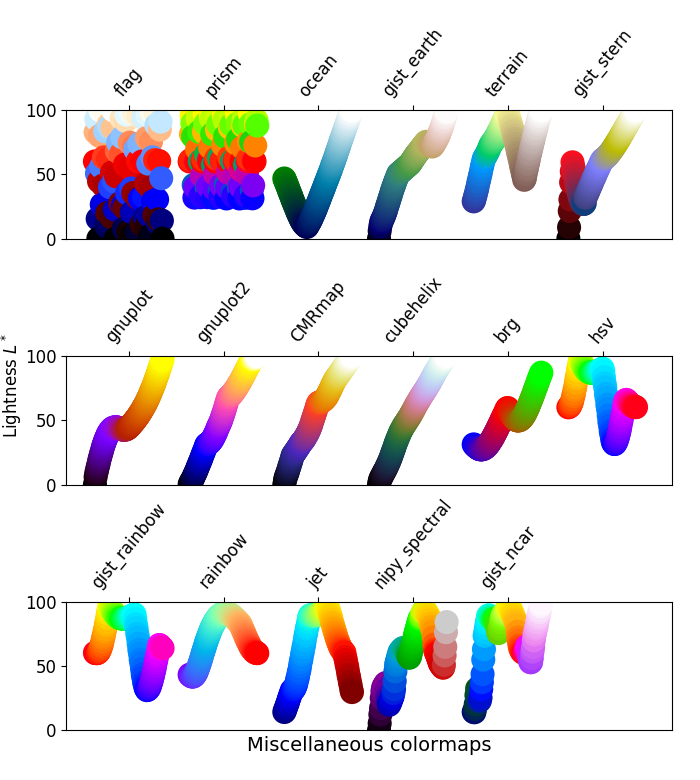# 线形图

Axes3D.plot 函数用来绘制线形图。

# line.py

import matplotlib.pyplot as plt
import numpy as np

from mpl_toolkits.mplot3d import Axes3D

fig = plt.figure()
ax = fig.gca(projection='3d')

x = np.linspace(-10, 10, 1000)
y = np.linspace(-10, 10, 1000)

ax.plot(x, y, z)
plt.show()


z轴取值为np.add(x, y)。请注意，np.add是元素级（element-wise）的运算：它是将x和y两个数组的元素逐个相加，所以得到的结果仍然是包含了1000个元素的数组。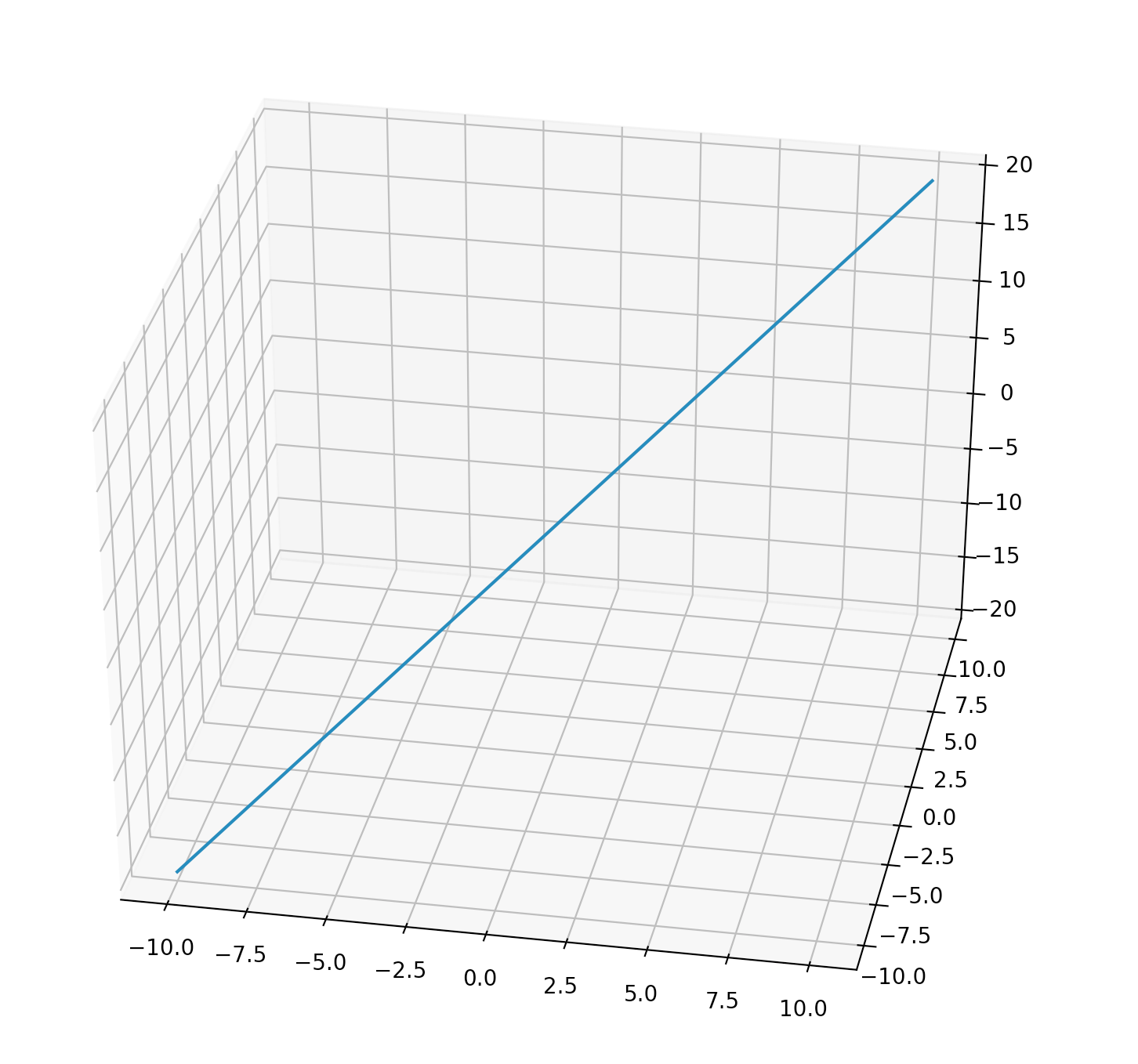# 散点图

Axes3D.scatter 函数用来绘制散点图。

# scatter.py

import matplotlib.pyplot as plt
import numpy as np

from mpl_toolkits.mplot3d import Axes3D

fig = plt.figure()
ax = fig.gca(projection='3d')

count = 100
range = 100

xs = np.random.rand(count) * range
ys = np.random.rand(count) * range
zs = np.random.rand(count) * range

ax.scatter(xs, ys, zs, s=zs, c=zs)

ax.set_xlabel('X Label')
ax.set_ylabel('Y Label')
ax.set_zlabel('Z Label')

plt.show()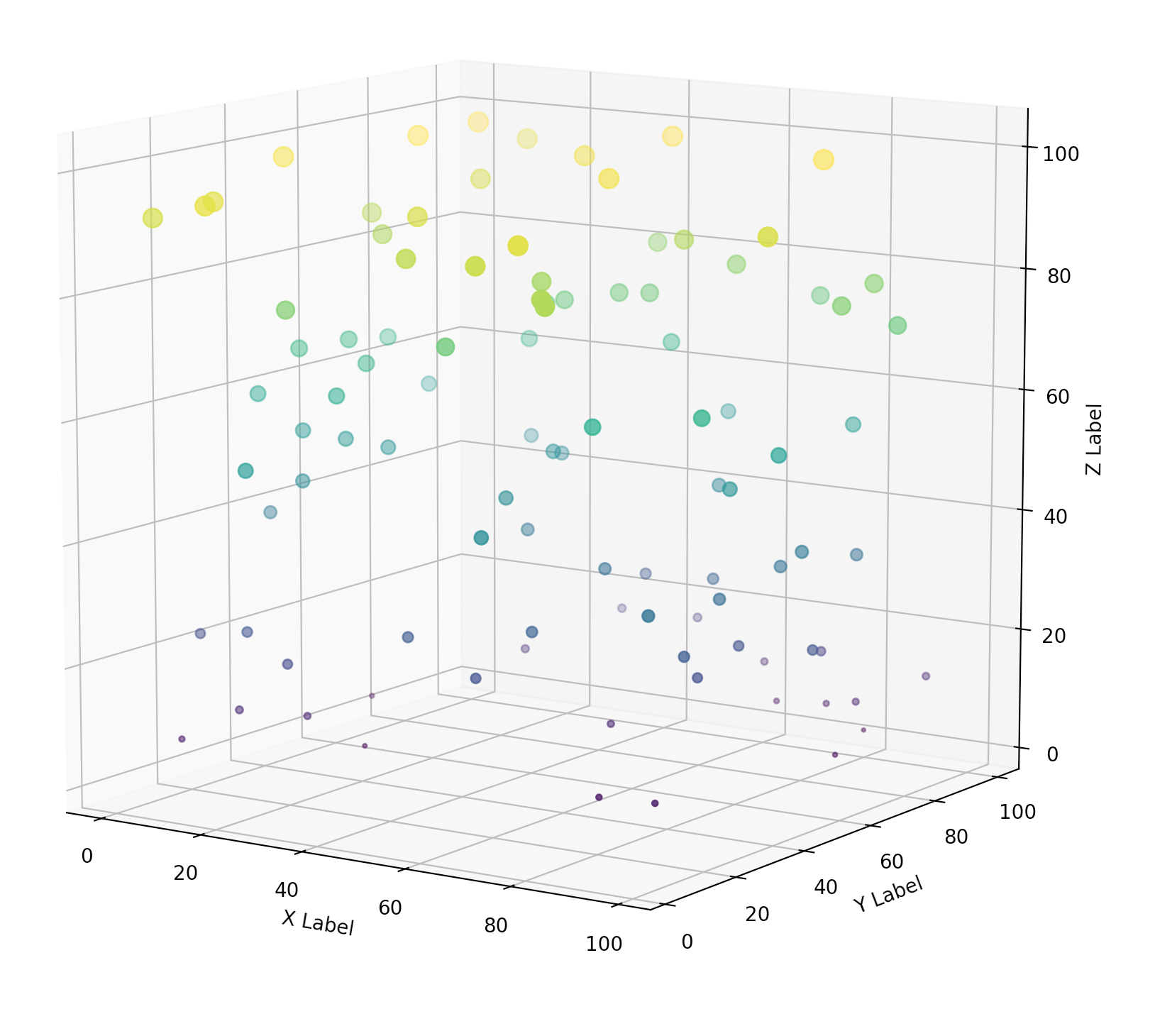# 线框图

Axes3D.plot_wireframe 函数用来绘制线框图。

$\begin{pmatrix} [1,11], [2,11], [3,11] \\\ [1,12], [2,12], [3,12] \\\ [1,13], [2,13], [3,13] \\\ [1,14], [2,14], [3,14] \\\ [1,15], [2,15], [3,15] \end{pmatrix}$

$\begin{pmatrix} 1, 2, 3 \\\ 1, 2, 3 \\\ 1, 2, 3 \\\ 1, 2, 3 \\\ 1, 2, 3 \end{pmatrix}$ $\begin{pmatrix} 11, 11, 11 \\\ 12, 12, 12 \\\ 13, 13, 13 \\\ 14, 14, 14 \\\ 15, 15, 15 \end{pmatrix}$

numpy中的meshgrid函数刚好可以帮我们完成这个功能，下面是一段代码示例：

# meshgrid_demo.py

import numpy as np

x = np.arange(1, 4)
y = np.arange(11, 16)
print(x)
print(y)

X, Y = np.meshgrid(x, y)
print(X)
print(Y)


[1 2 3]
[11 12 13 14 15]
[[1 2 3]
[1 2 3]
[1 2 3]
[1 2 3]
[1 2 3]]
[[11 11 11]
[12 12 12]
[13 13 13]
[14 14 14]
[15 15 15]]


$z = -x^3 + y^4 , -10 \lt x,y \lt 10$

# wireframe.py

import matplotlib.pyplot as plt
import numpy as np

from mpl_toolkits.mplot3d import Axes3D

fig = plt.figure()
ax = fig.gca(projection='3d')

x = np.arange(-10, 10, 0.1)
y = np.arange(-10, 10, 0.1)
X, Y = np.meshgrid(x, y)

Z = np.add(-np.power(X, 3), np.power(Y, 4))

surf = ax.plot_wireframe(X, Y, Z)

plt.show()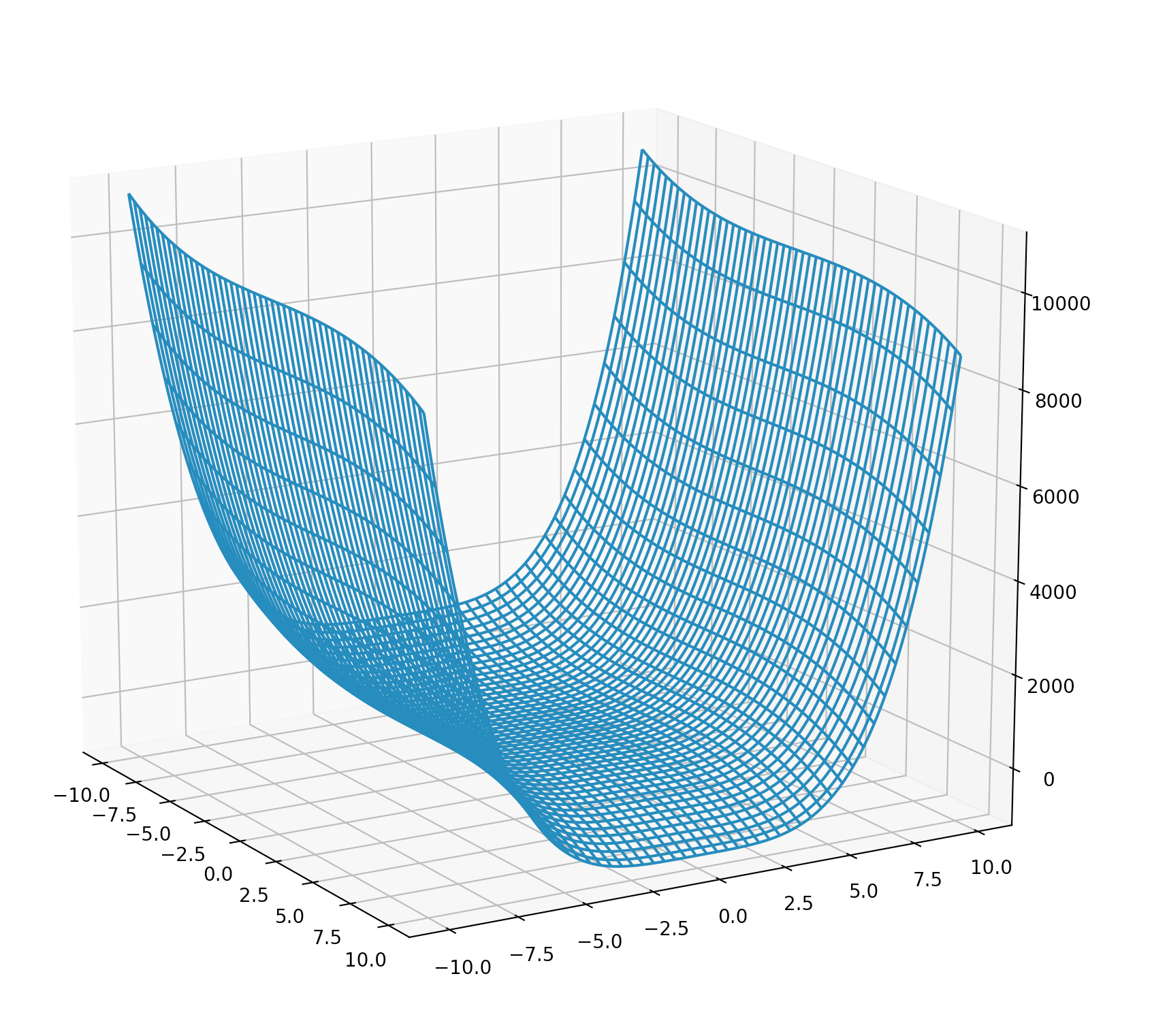# 曲面图

Axes3D.plot_surface 函数用来绘制曲面图。

$z = -x^3 + y^2, -10 \lt x, y \lt 10$
# surface.py

import matplotlib.pyplot as plt
import numpy as np

from matplotlib import cm
from mpl_toolkits.mplot3d import Axes3D

fig = plt.figure()
ax = fig.gca(projection='3d')

x = np.arange(-10, 10, 0.1)
y = np.arange(-10, 10, 0.1)
X, Y = np.meshgrid(x, y)

Z = np.add(-np.power(X, 3), np.power(Y, 2))

surf = ax.plot_surface(X, Y, Z, cmap=cm.gist_rainbow)
fig.colorbar(surf, shrink=0.5, aspect=5)

plt.show()


1. 这里通过cmap=cm.gist_rainbow指定了曲面的颜色。更多的Colormap请到查阅这里：Choosing Colormaps
2. 通过 fig.colorbar(surf, shrink=0.5, aspect=5)添加了一个色彩条。shrink指定了色彩条与图形高度的比例，aspect指定了色彩条本身的长宽比。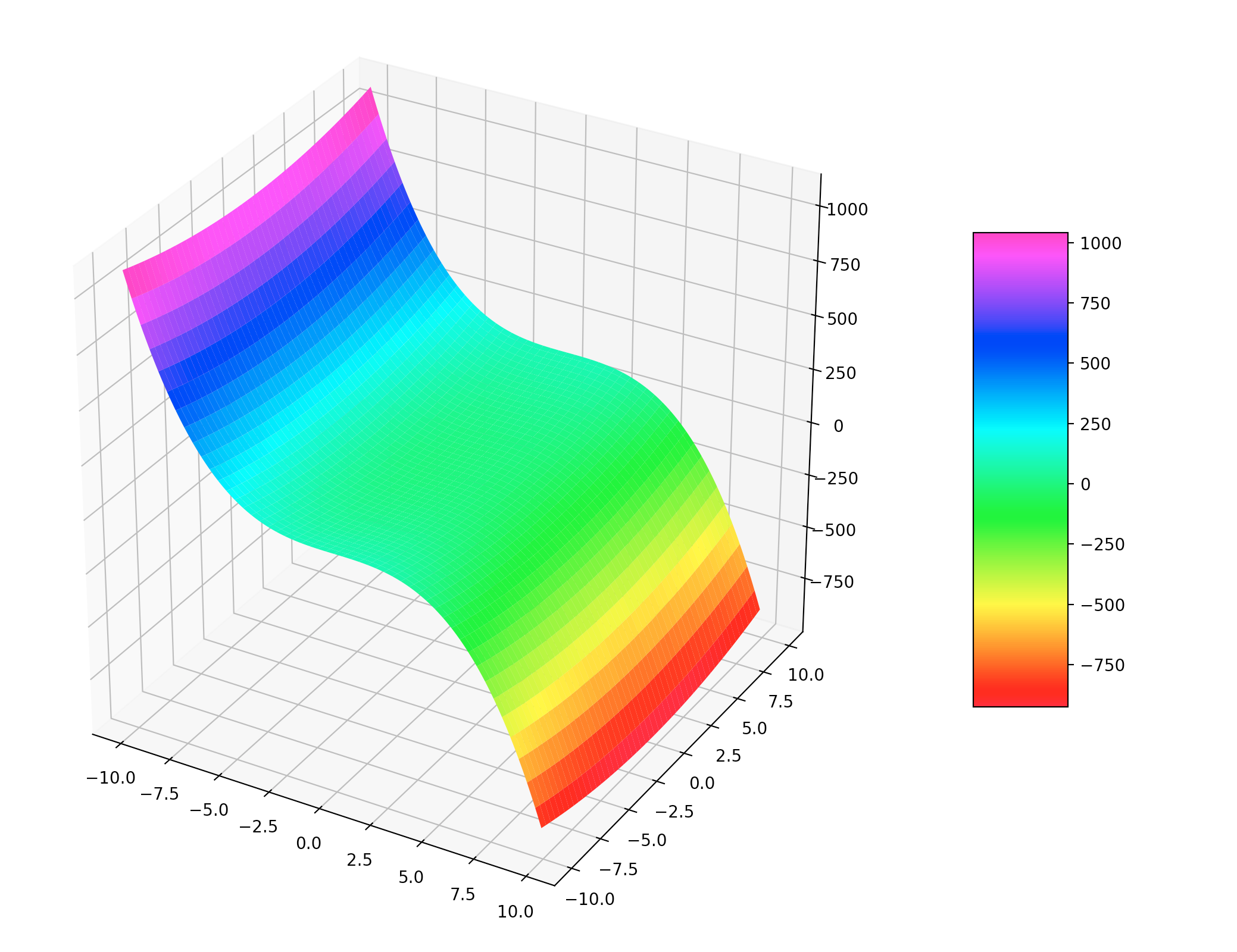# 等高线

Axes3D.contour 函数用来绘制等高线。

$Z = -x^4 + Y^4, -10 \lt x, y \lt 10$
# contour.py

import matplotlib.pyplot as plt
import numpy as np

from matplotlib import cm
from mpl_toolkits.mplot3d import Axes3D

fig = plt.figure()
ax = fig.gca(projection='3d')

x = np.arange(-10, 10, 0.1)
y = np.arange(-10, 10, 0.1)
X, Y = np.meshgrid(x, y)

Z = np.add(-np.power(X, 4), np.power(Y, 4))

ax.set_xlabel("X")
ax.set_ylabel("Y")
ax.plot_wireframe(X, Y, Z, alpha=0.1)
ax.contour(X, Y, Z, cmap=cm.Accent, linewidths=2)

plt.show()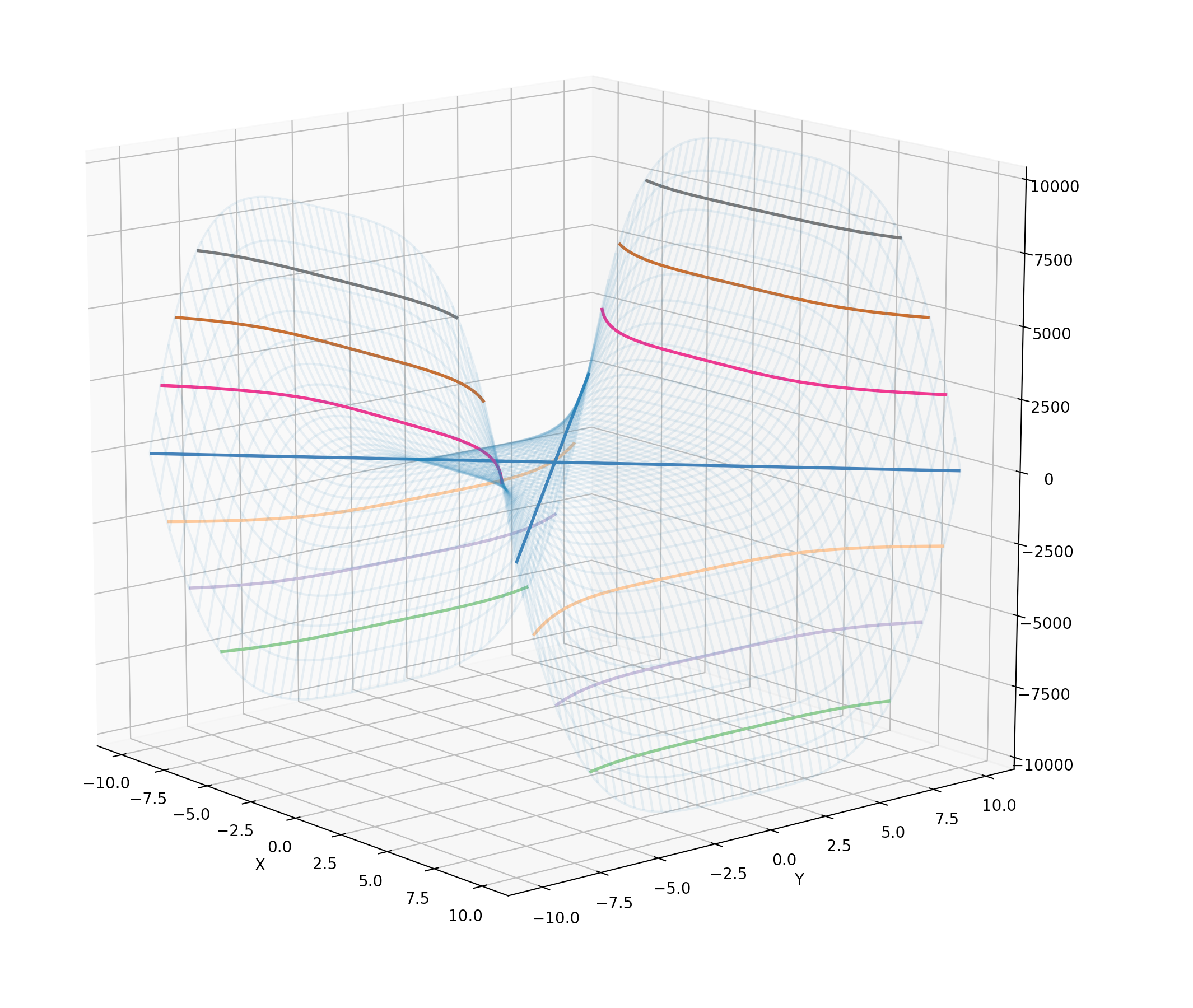# 柱状图

Axes3D.bar 函数用来绘制柱状图。

# bar.py

import matplotlib.pyplot as plt
import numpy as np

from matplotlib.collections import PolyCollection
from mpl_toolkits.mplot3d import Axes3D

fig = plt.figure()
ax = fig.gca(projection='3d')

np.random.seed(59)
month = np.arange(1, 12)
years = [2016, 2017, 2018, 2019]

def get_color(value_array):
color = []
for v in value_array:
if (v < 50):
color.append('y')
elif (v < 100):
color.append('g')
elif (v < 150):
color.append('b')
elif (v < 200):
color.append('c')
elif (v < 250):
color.append('m')
else:
color.append('r')
return color

for year, c in zip(years, ['b','c','r','m']):
value = np.random.rand(len(month)) * 300
ax.bar(month, value, year, zdir='y', color=get_color(value), alpha=0.7)
for i in np.arange(0, 12):
ax.bar

ax.set_xlabel('Month')
ax.set_xticks(np.arange(1, 13))
ax.set_ylabel('Year')
ax.set_yticks(np.arange(2016, 2020))
ax.set_zlabel('Precipitation')

plt.show()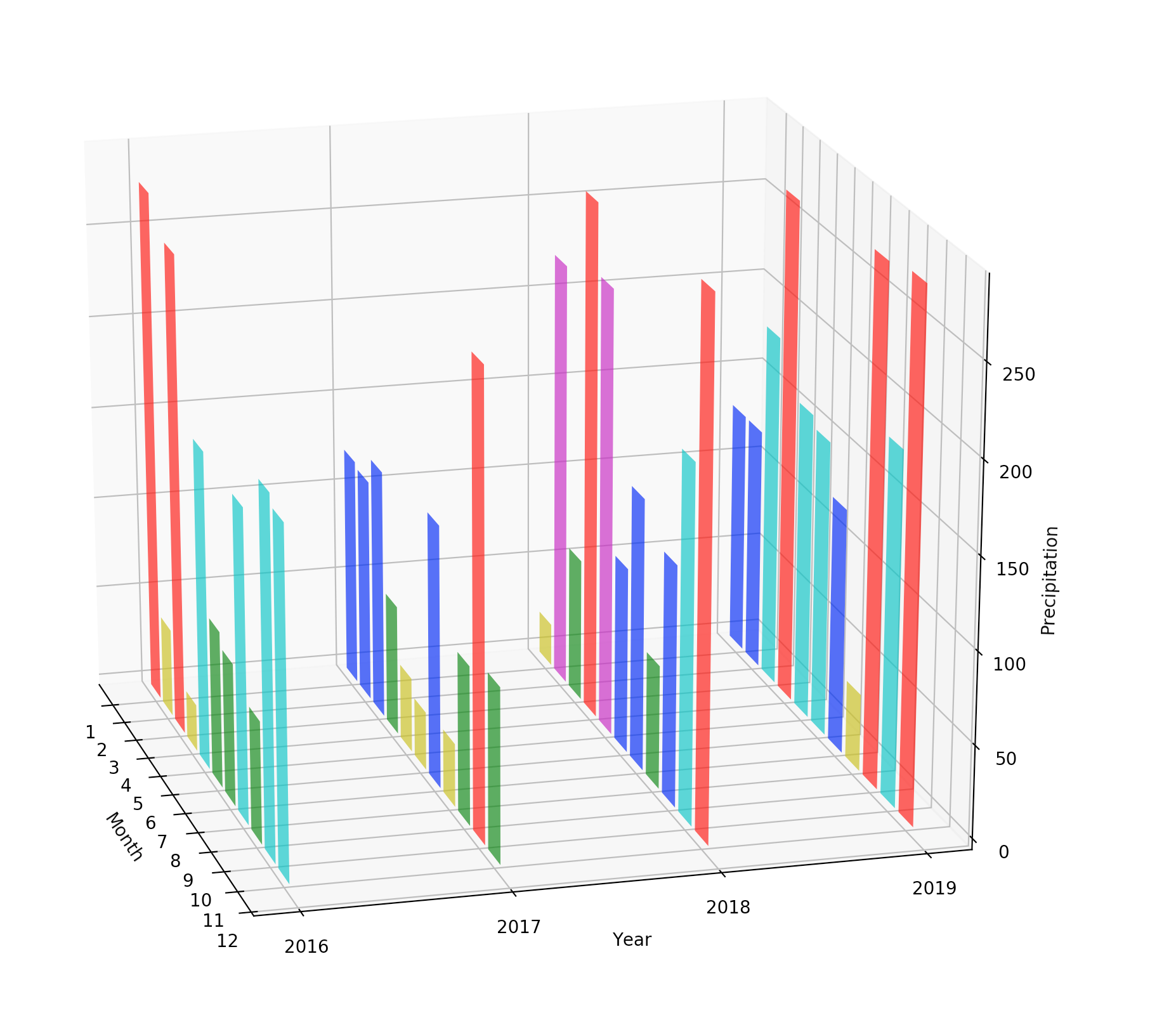# 多边形

# poly.py

import matplotlib.pyplot as plt
import numpy as np

from matplotlib.collections import PolyCollection
from mpl_toolkits.mplot3d import Axes3D

fig = plt.figure()
ax = fig.gca(projection='3d')

np.random.seed(59)
month = np.arange(0, 13)
years = [2016, 2017, 2018, 2019]

precipitation = []
for year in years:
value = np.random.rand(len(month)) * 300
value, value[-1] = 0, 0
precipitation.append(list(zip(month, value)))

poly = PolyCollection(precipitation, facecolors=['b','c','r','m'])
poly.set_alpha(0.7)

ax.set_xlabel('Month')
ax.set_xlim3d(0, 12)
ax.set_ylabel('Year')
ax.set_ylim3d(2015, 2020)
ax.set_zlabel('Precipitation')
ax.set_zlim3d(0, 300)

plt.show()


Axes3D.add_collection3d 函数除了支持PolyCollection，还支持LineCollectionPatchCollection。这一点，读者可以自行研究一下。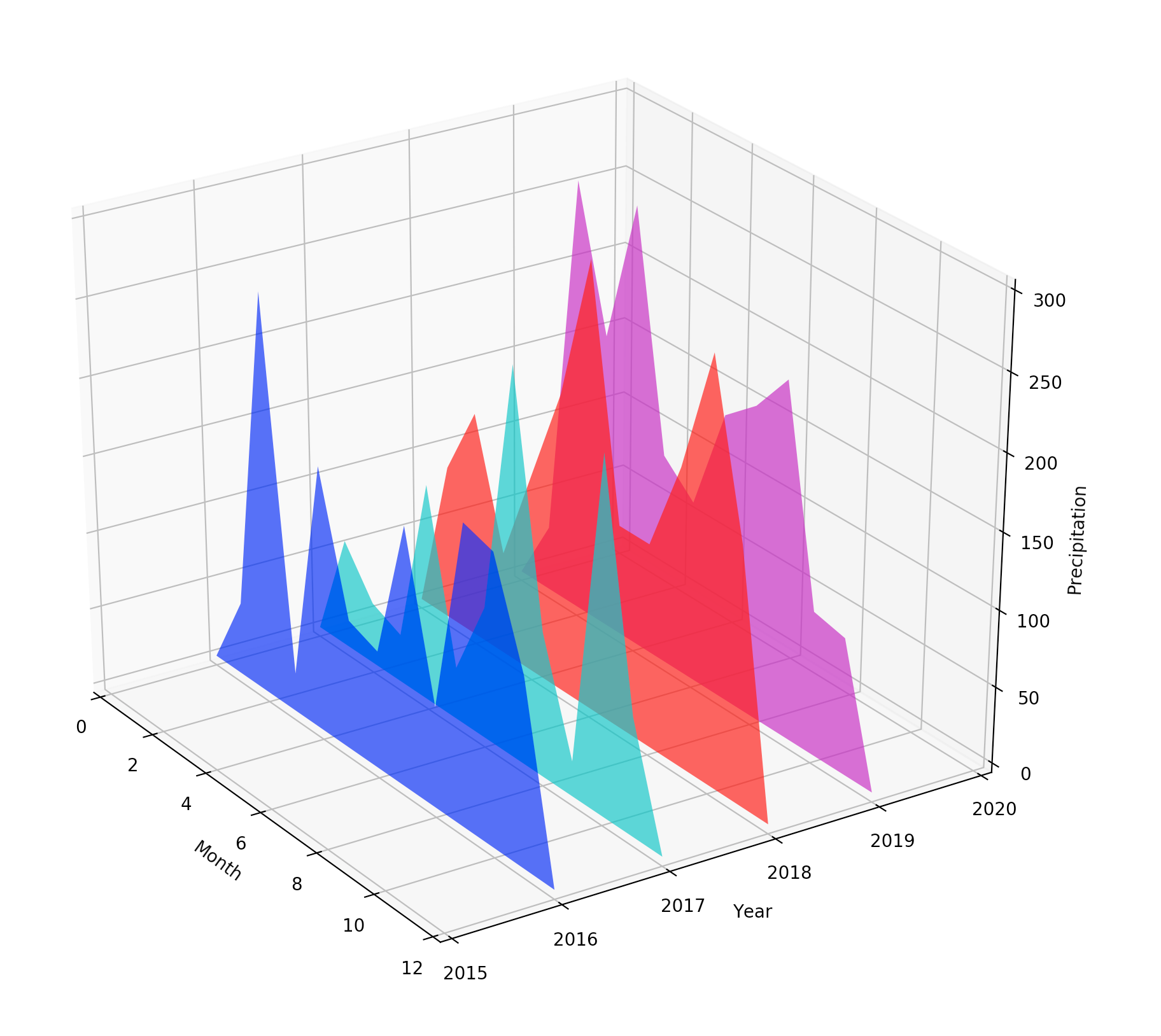# 制作动图

## 生成不同角度的图形

# surface_files.py

import matplotlib.pyplot as plt
import numpy as np

from matplotlib import cm
from mpl_toolkits.mplot3d import Axes3D

fig = plt.figure(figsize=(10, 8))
ax = fig.gca(projection='3d')

x = np.arange(-10, 10, 0.1)
y = np.arange(-10, 10, 0.1)
X, Y = np.meshgrid(x, y)

Z = np.add(-np.power(X, 4), np.power(Y, 4))

ax.set_xlabel("X")
ax.set_ylabel("Y")
ax.plot_surface(X, Y, Z, cmap=cm.hsv)

for angle in range(95, 180, 3):
ax.set_zlabel("Angle: " + str(angle))
ax.view_init(30, angle)
filename = "./" + str(angle) + ".png"
plt.savefig(filename)
print("Save " + filename + " finish")


• 通过set_zlabel设置了当前旋转角度的Label
• 通过ax.view_init(30, angle)设置图形的视角
• 根据当前角度生成一个单独的文件名称
• 通过plt.savefig(filename)保存文件
• 打印日志

## 使用ImageMagick

brew install ImageMagick


convert -delay 50 *.png animated.gif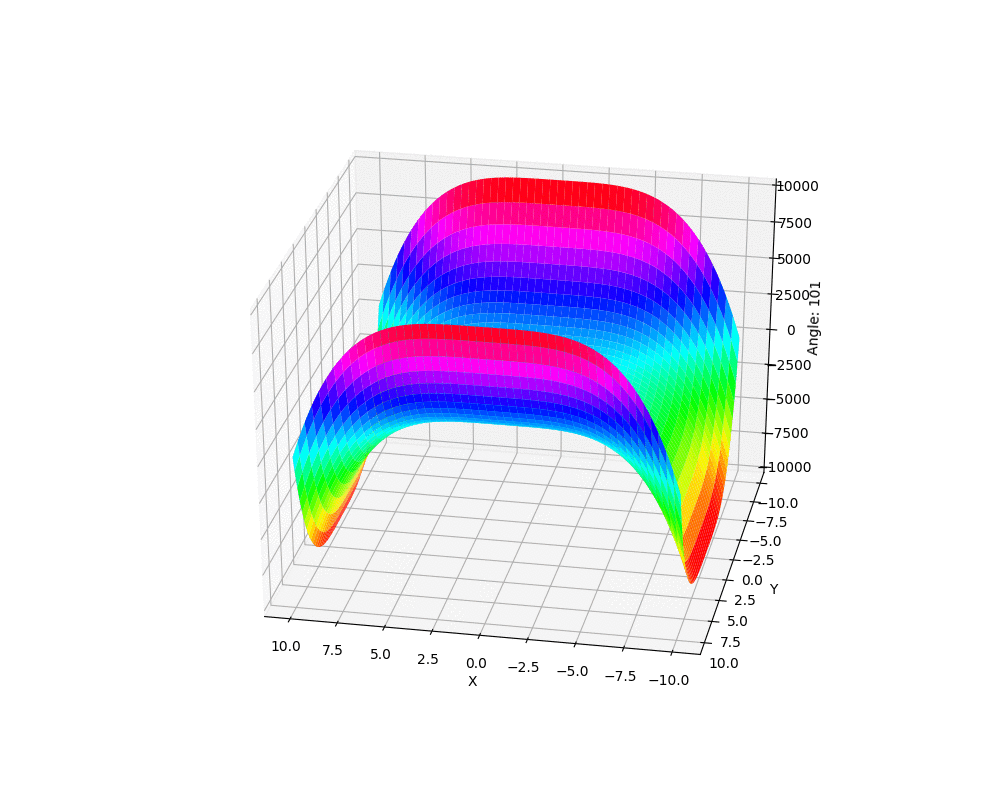Contents